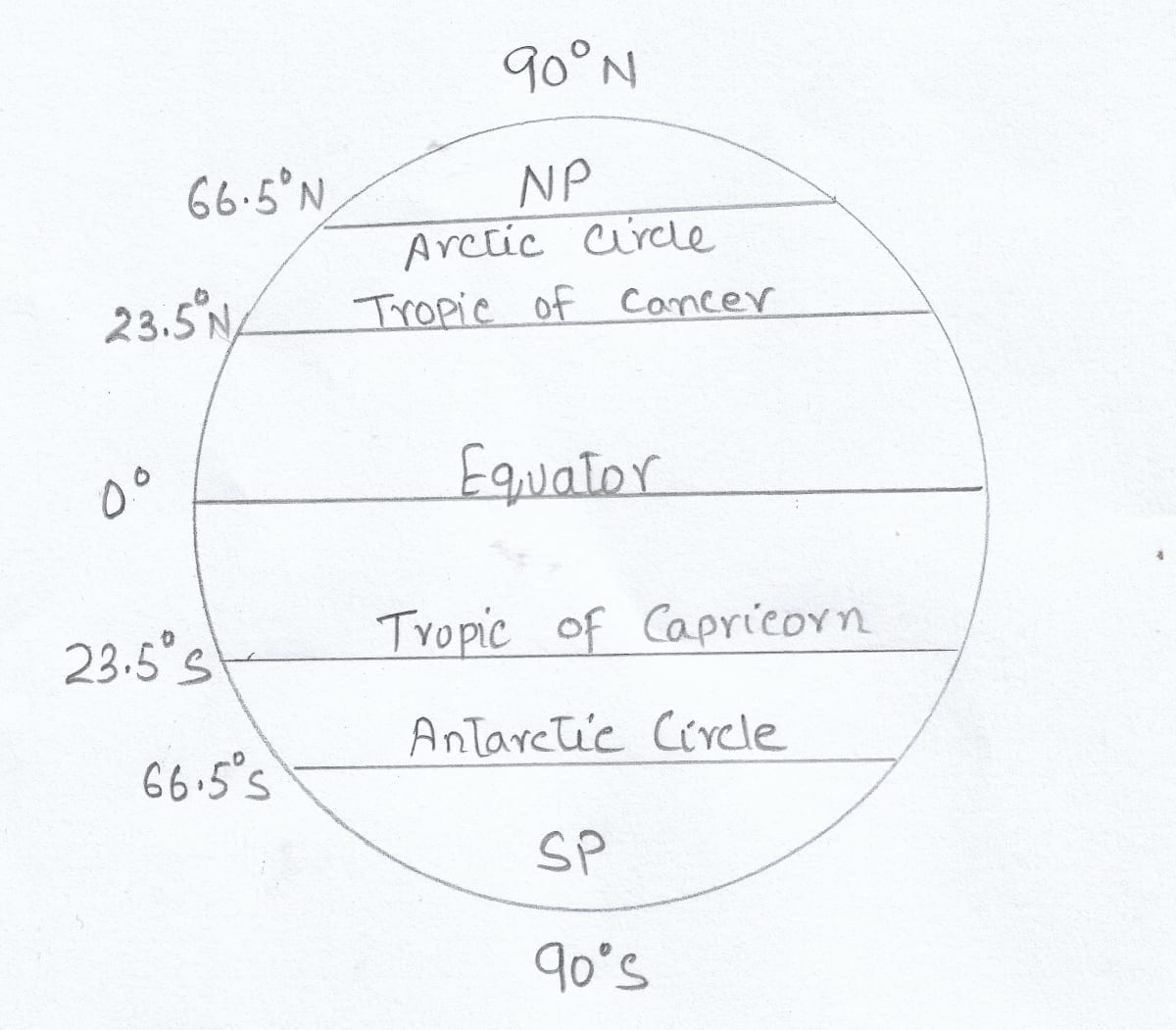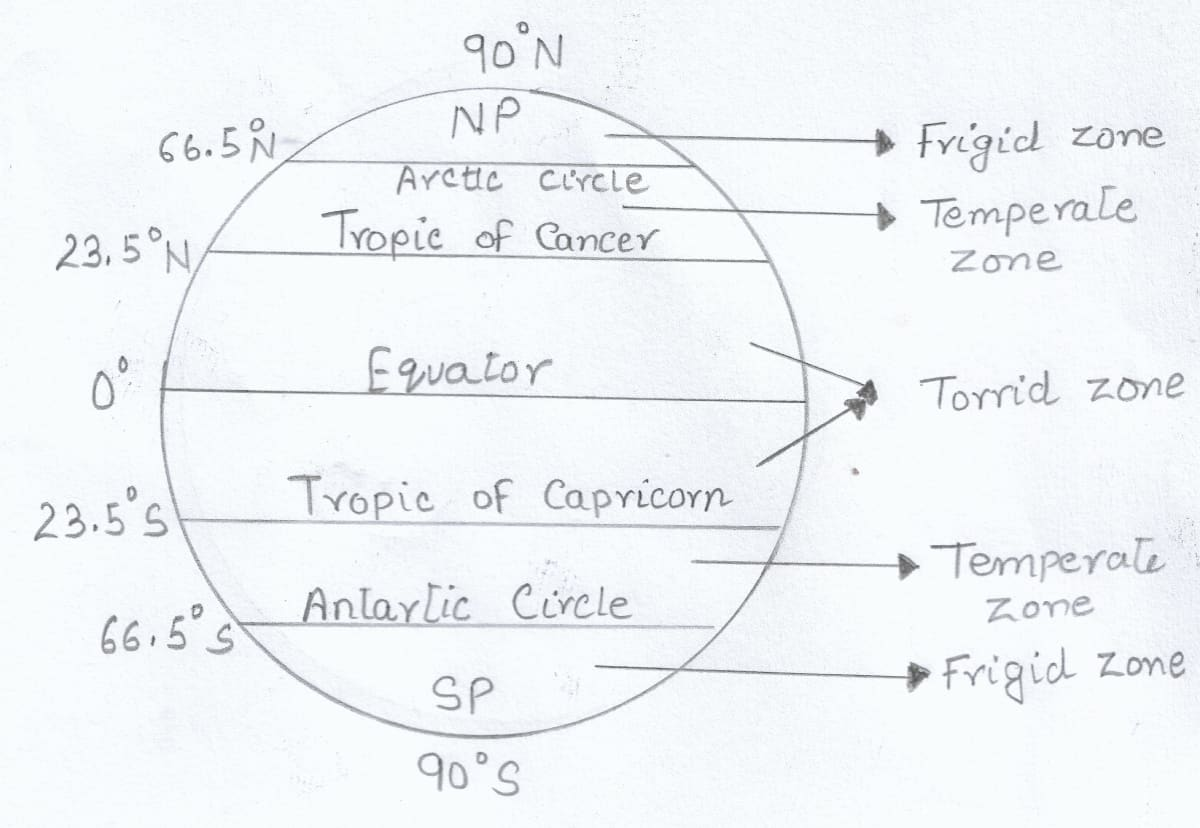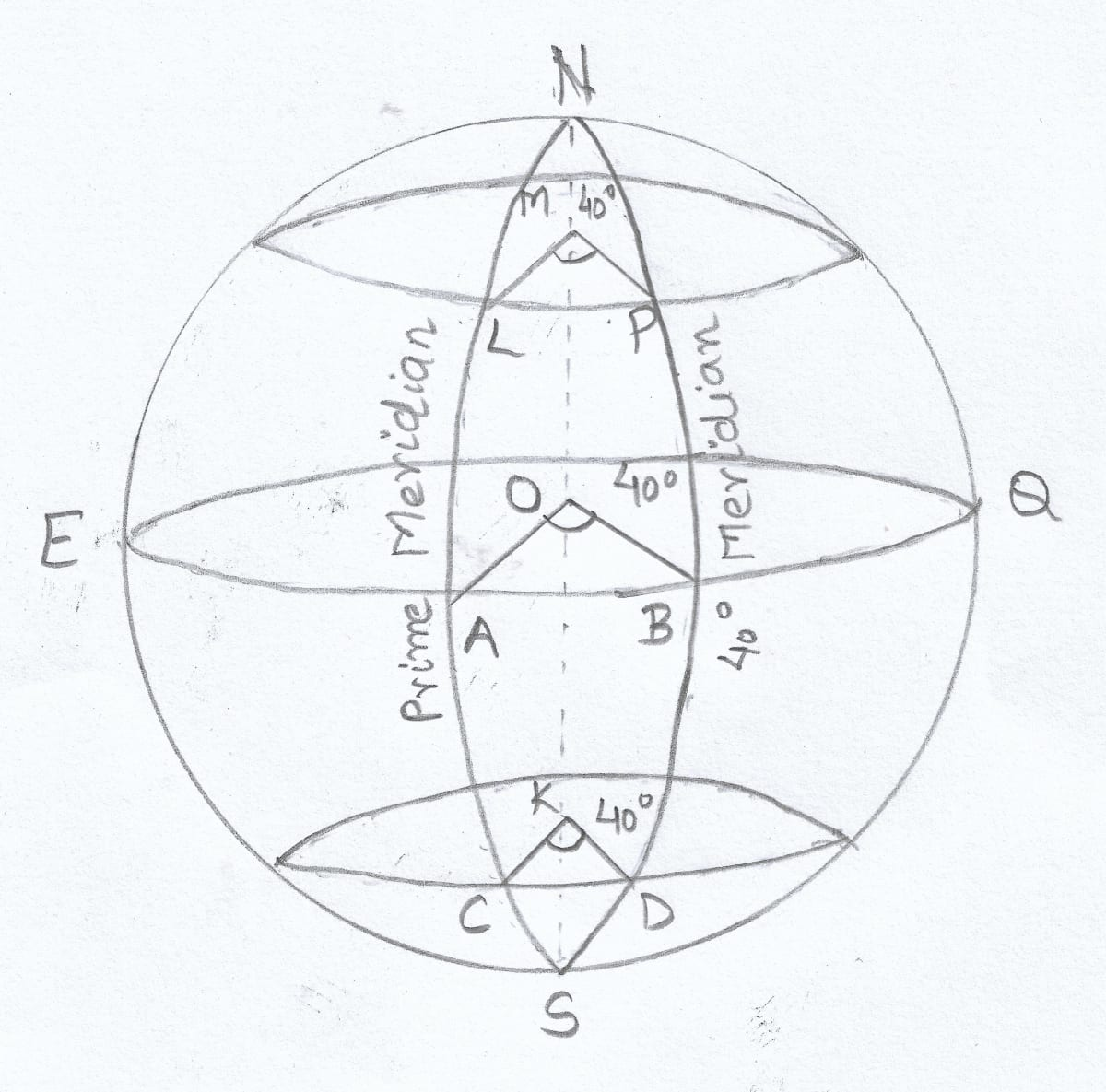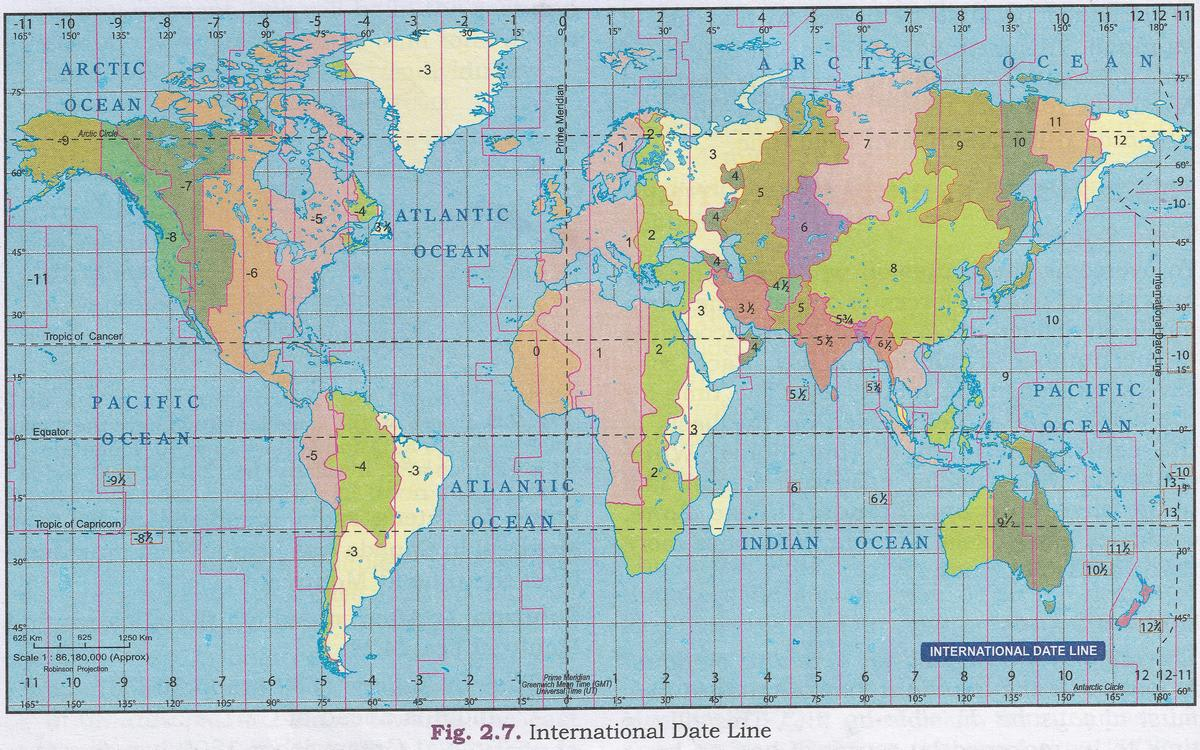# Geographic Grid: Latitudes and Longitudes

## Short Answer Questions

#### Question 1

What is a geographic grid?

A framework of lines of longitude and latitude on a globe or a map is called a geographic grid.

#### Question 2

Who devised the lines of latitude and longitude?

Eratosthenes, the Greek philosopher, devised the lines of latitude and longitude

#### Question 3

What are lines of latitude and longitude?

The imaginary lines running east to west, parallel to the equator are called lines of latitude.

The imaginary lines running north to south passing through the poles are called lines of longitude.

#### Question 4

Mention two characteristics of lines of latitude.

Two characteristics of lines of latitude are:

1. Lines of latitude are imaginary lines joining all places having the same latitude towards north or south of the equator.
2. A latitude is marked in degrees, with equator being 0 degrees.

#### Question 5

Name the two hemispheres of the earth made by the Equator.

The two hemispheres of the earth made by the Equator are-

1. Northern hemisphere
2. Southern hemisphere

#### Question 6

Express 1° angular distance in kilometres.

1° angular distance is equal to 111 kilometres.

#### Question 7

Name the thermal zones of the earth.

The thermal zones of the earth are-

1. Torrid or Tropical zone
2. Temperate zone
3. Frigid zone

#### Question 8

With the help of a diagram, show the important lines of latitude.#### Question 9

Which temperature zone receives almost vertical rays of the sun and which zones receive slanting rays?

Torrid zone receives almost vertical rays of the sun and temperate zone and frigid zone receive slanting rays of the sun.

#### Question 10

Which line is known as the Prime Meridian? State its importance.

The Prime Meridian is the line of longitude whose angular distance is defined as 0 degrees. It passes through Greenwich near London.

While fixing the Time Zones, the time at Prime Meridian has been selected as the mean time. The time for all the other places is calculated with reference to the time at Prime Meridian.

#### Question 11

How can the general climate of an area be described with the help of the lines of latitude?

Lines of latitude divide the earth into three distinct heat belts -

1. Torrid zone, being the hottest zone
2. Temperate zone with moderate climate
3. Frigid zone with the coldest climate

The lines of latitude indicate the general climate of the area by applying the principle of heat zones of the earth.

#### Question 12

Which line of longitude is used to fix the World Standard Time? State its value in degrees.

Prime Meridian is used to fix the World Standard Time. Its value in degrees is 0°.

#### Question 13

State the longitudinal value in degrees of Indian Standard Meridian.

The longitudinal value of Indian Standard Meridian is 82° 30'E.

#### Question 14

What are the Great Circle Routes? State their importance.

A great circle route is the shortest distance between two places on the earth and lies on the arc of a great circle.

Great Circle Routes are specially important for places that are on opposite sides of the globe.

## Structured Questions

#### Question 1(a)

State the main five lines of latitude.

The main five lines of latitude are-

1. Equator (0°)
2. The Tropic of Cancer (23.5° N)
3. The Tropic of Capricorn (23.5° S)
4. The Arctic circle (66.5° N)
5. The Antarctic circle (66.5° S)

#### Question 1(b)

What is the significance of these lines of latitude?

These lines of latitude form one of the coordinates of the grid system. The lines of latitude have the following significance-

1. They are used to find the location of a place
2. They are used to measure the distance of a place
3. They divide the earth into distinct heat zones

#### Question 1(c)

Give a geographical reason for each of the following:
(i) Lines of latitude are called parallels of latitude.
(ii) Lines of latitude are not of equal length.
(iii) Lines of latitude carve out the heat zones of the earth.

(i) Lines of latitude are called parallels of latitude as these lines are parallel to the Equator and each other.

(ii) Lines of latitude are not of equal length as they become smaller as we move towards the poles.

(iii) Lines of latitude carve out the heat zones of the earth as the temperature goes on decreasing from the equator towards the poles. Thus, these lines indicate the general climate of an area by applying the principle of heat zones.

#### Question 1(d)

Draw a well labelled diagram showing the different heat zones of the world.#### Question 2(a)

Describe the lines of longitude.

The imaginary lines running north to south passing through the poles are called lines of longitude.

#### Question 2(b)

State the use of the lines of longitude in relation to distance and time.

The earth makes a complete circle on its axis covering 360° in 24 hours. It covers 1° longitude every 4 minutes. For each 1° longitude towards the east, 4 minutes are to be added and for each 1° longitude towards the west, 4 minutes are to be subtracted.

#### Question 2(c)

Give a geographical reason for each of the following:
(i) Lines of longitude are called meridians of longitude.
(ii) Lines of longitude are of same length.
(iii) Diametrically opposite lines of longitude and the Equator are called Great Circles.

(i) Lines of longitude are called meridians of longitude because the sun crosses a meridian at noon. So, all places on a particular meridian will have noon at the same time.

(ii) Lines of longitude are of same length as they all converge at the poles.

(iii) Diametrically opposite lines of longitude and the Equator are called Great Circles because they pass through or touch the centre of the circle. Also, they have a radius equal to the radius of the sphere's centre.

#### Question 2(d)

Draw a well labelled diagram to show that places on the same longitude have the same time.#### Question 3(a)

State the meaning of the International Date Line.

The line of longitude 180° is one and the same for east or west of the Prime Meridian. Since it is diametrically opposite to the Greenwich Meridian, it causes a time difference of a full day on crossing the line.

The International Date Line is the 180° line. When crossing this date line from the west to east a day is deducted or the time is fixed backward by one day. When crossing the International Date Line, from east to west, a day is added.

#### Question 3(b)

State with an example how time lost or gained is computed with reference to the International Date Line.

The International Date Line is the 180° line. When crossing this date line from the west to east a day is deducted or the time is fixed backward by one day. When crossing the International Date Line, from east to west, a day is added.

For example, if it is 8 pm Monday 25th December at Greenwich, it will be 8 am Tuesday, 26th December on crossing the 180° line from the east as time will be 12 hours ahead. But if one were to travel from west, it will be 12 hours behind or 8 am Monday, 25th December.

#### Question 3(c)

Give a geographical reason for each of the following:
(i) The International Date Line deviates and goes zig-zag near some islands in the Pacific Ocean.
(ii) The Greenwich time is called the Greenwich Mean Time.
(iii) Great Circles are the shortest routes between two places.

(i) The International Date Line deviates and goes zig-zag near some islands in the Pacific Ocean to avoid the confusion of having different dates within the same country.

(ii) The Greenwich time is called the Greenwich Mean Time as while fixing the time zones, the time at Greenwich has been selected as the mean time and provides the basis for calculating the Standard Time of other places.

(iii) Great Circles are the shortest routes between two places as we can connect any two places on the earth's surface by the curvature line of the great circle. And this curvature is the smallest possible route between those two places, because this curvature directly connects those places or points.

#### Question 3(d)

State with one practical example how is time of a place found with the help of longitudes.

As the earth takes 24 hours to make a complete rotation along its axis crossing 360° lines of longitude, so earth takes nearly 4 minutes to move 1° longitude.

For example, Indian Standard Time is based on 82 1/2° E longitude. So if the time in India is 12 noon, so it will be 6.30 A.M. in England or GMT.

The time difference between 0° and 82 1/2° E will be-
82.5 × 4 = 330 minutes or 330 ÷ 60 = 5 1/2 hours

So there is difference of 5 1/2 hours between India and England, as England lies in the west, so the time will be 5 1/2 hours less than India.

## Project/Activity

Look at the map of the Time Zones (Fig. 2.7) and answer the following questions:#### Question 1

What is the Longitudinal degree of IST?

82 1/2° E

#### Question 2

If it is 2 a.m. IST, what will be the Time at Tokyo, London, San Francisco?

Tokyo - 5:30 am

London - 9:30 pm

San Francisco - 1:30 pm

#### Question 3

Name the place which is diametrically opposite of India.